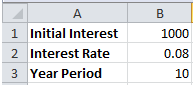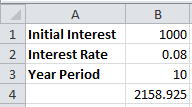## How to calculate compound interest in Excel?

If you have a bank account which may have its interest compounded every year, and ten years later, how much total interest can you get from your account? In this case, I talk about how to calculate the compound interest in Excel.

Compound interest arises when interest is added to the principal of a deposit or loan, so that, from that moment on, the interest that has been added also earns interest.

Calculate compound interest by formula in Excel

Calculate compound interest by Function in Excel

####Calculate compound interest by formula in Excel###### Save 50% of your time, and reduce thousands of mouse clicks for you every day!

In Excel, here is a formula that can help you to quickly calculate the compound interest.

Supposing there is \$1000 initial principal in your account and the interest rate is 8% per year, and you want to calculate the total interest in ten years later.

Select a blank cell, and type this formula =1000*(1+0.08)^10 into it, then click Enter button on the keyboard, you will get the total compound interest.

Tip: In the above formula, 1000 indicates the initial principal of your account, 0.08 stands the interest rate every year, 10 is the number of account investment periods, and you can change them as you need.

####Calculate compound interest by Function in Excel

In addition to the formula, you also can use Function to calculate the compound interest.

Supposing there is \$1000 initial principal in your account with 8% interest rate per year, and you want to calculate the total interest in ten years later.

1. Type the initial principal data, interest rate, and period into cells, in this instance, I type them to B1, B2 and B3 separately. See screenshot:2. Hold ALT button and press F11 on the keyboard to open a Microsoft Visual Basic for Application window.

3. Click Insert > Module, and copy the VBA into the module.

VBA: Calculate compound interest

``````Function YearlyRate(pMoney As Double, pRate As Double, pTime As Double) As Double
'Updateby20140321
YearlyRate = pMoney * (1 + pRate) ^ pTime
End Function
``````

4. Save the code and close the window, then in a blank cell, for instance, the Cell B4, type =YearlyRate(B1,B2,B3) (B1 indicates initial principal, B2 is interest rate, B3 stands the number of periods, you can change them as you need), then press the Enter key. See screenshot: